ChemTalk

# Boyle’s Law## Boyle’s Law Core Concepts

In this tutorial, you will learn about the Boyle’s law formula, and how this equation can be used to determine relationships between changes in pressure and changes in volume in a given closed system.

Robert Boyle, sometimes called the “Father of Modern Chemistry” worked in the fields of physics and chemistry. In 1660, Boyle published The Spring and Weight of the Air in which he described different experiments he created using a vacuum pump which he designed.

In 1662, Boyle published version two of The Spring and Weight of the Air. This is were he described the inverse relationship between pressure and volume, now known as Boyle’s Law. Boyle’s Law states that pressure and volume are inversely proportional to each other. As the pressure increases the volume decreases, and as the pressure decreases the volume increases. He made these observations by using mercury in a J-tube, and then made measurements of the volume of the gas at pressures both lower and higher than standard atmospheric pressure.

Boyle expressed his results in a relationship that is known as Boyle’s law equation or Boyle’s law formula: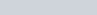. This law assumes the temperature remains constant.

## Important Things to Consider

The gas law described in this article only applies to ideal gases, which you can read about on our article, The Ideal Gas Law. Under nonideal conditions, such as high pressures and low temperatures, repulsive or attractive forces between gas particles results in pressure and volume not having as close of a relationship. Specifically, too much attractive force results in a gas occupying a smaller volume at a given pressure than Boyle’s Law would suggest. Conversely, too much repulsive force results in a larger volume.

## Relationship Between Pressure and Volume

Consider a sample of gas in a 1-liter container. From our article, What is Pressure, we know that the pressure exerted on the container from the gas is the sum of the collisions of the particles, divided by the surface area of the container,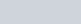. We also know that the volume is related to the surface area, and if the volume decreases, the surface area will decrease as well.

From these two relations, we can see that as the volume decreases, the total pressure is going to increase. This leads us to Boyle’s law formula,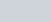.

We can make a graph of this relationship as follows:

## Change in Pressure and Volume

This proportionality can enable us to solve specific problems relating to the changes in pressure and volume in a closed system.

Consider, for example, a piston full of oxygen. From this proportionality, we know that if the piston is compressed, the pressure of the gas will increase.

## Boyle’s Law Equation – Example Problem

Here is an example of how you can solve a Boyle’s Law problem.

An ideal gas exerts a pressure of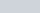in a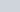container. What will the pressure conequently be if the volume of the container is changed to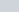at constant temperature?

Solution:You can read about how a student used Boyle’s original data to verify Boyle’s Law.

## Boyle’s Law Practice Problems

Problem 1

Inside a piston, a gas occupies the full volume of the piston at a pressure of. You then compress the gas until it occupies a third of the piston’s volume. Calculate the gas’s final pressure after the compression.

Problem 2

You have a sample of gas within Container A. Firstly, you transfer the gas completely into Container B and notice that the gas’s pressure decreases. Secondly, you transfer the gas completely into Container C and notice the pressure is higher than when it was in Container A. Thirdly, you transfer half of the gas into Container D and notice the pressure is equal to its pressure in Container C. Rank each container from largest to smallest volume.

## Boyle’s Law Practice Problem Solutions

1: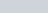2: B > A > C > D